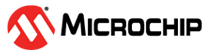• All
• Products
• Documents
• Applications Notes

# Floating Point Math Library for PIC24 and dsPIC

 Microchip’s ANSI C compiler provides full floating point math capability, enabling both the single and double precision floating point operations found in any standard ANSI C compiler. These include functions such as sin, cos, tan, and other common mathematical operations. Description The standard ANSI C floating point library is part of the MPLAB compiler and is documented in the 16-bit Language Tools document. The library works with both the PIC24 and dsPIC families. • Compatible with the Microchip PIC24and dsPIC families • Full compatibility with standard ANSI C floating point math libraries. • Support for double or single precision floating point • Includes common operations such as sin, cos, tan, etc. Devices Supported All processors in the PIC24and dsPIC DSC family Getting Started • Download and install MPLAB and the free trial version of the C compiler • See Chapter 3 of the 16-bit Language Tools for complete documentation of the Floating Point Math Library • Start writing floating point code! Common Floating Point Math Functions Function Description sin/sinf* Sin of a double/single precision floating point variable cos/cosf* Cos of a double/single precision floating point variable tan/tanf* Tan of a double/single precision floating point variable exp/expf* Calculates the exponential function of a double/single precision floating point variable fabs/fabsf* Calculates the absolute value of a double/single precision floating point variable fmod/fmodf* Calculates the remainder of x/y as a double/single precision floating point value log/logf* Calculates the log of a double/single precision variable sqrt/sqrtf* Calculates the square root of a double/single precision variable pow/powf* Calculates x raised to the power of y floor/floorf* Calculates the floor of a double/single precision floating point variable ceil/ceilf* Calculates the ceiling of a double/single precision floating point variable asin/asinf* Arc sin of a double/single precision floating point variable acos/acosf* Arc cos of a double/single precision floating point variable atan/atanf* Arc tan of a double/single precision floating point variable *- “f” denotes single precision floating point number A complete list of floating point math functions is available in the16-Bit Language Tools document